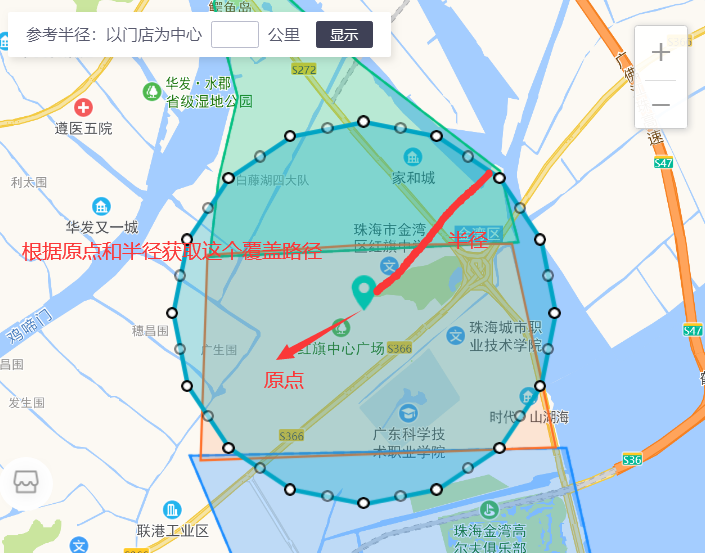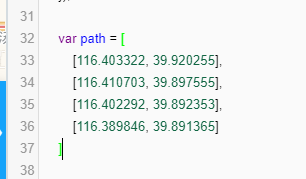2021-03-02 18:28

# 求救，高德地图，近似圆的多边形怎么画• 点赞
• 收藏

#### 3条回答默认 最新

•ProfSnail 2021-03-02 18:44
已采纳

思路是根据圆心的经纬度和半径，遍历差值为2pi/n的角度，获取最后的路径点。

具体实现交给题主自己来吧，还是需要花点时间研究一下怎么经纬转换的。

点赞 评论
•Hobart-Ljw 2021-03-03 11:15

// 根据坐标、半径获取圆切点

getPolygonPath () {

// 坐标点

let centerpoint = {

longitude: 113.376778,

latitude: 23.13588

}

let radius = 10000 // 半径

let r = 6371000.79 // 相同体积的球半径R3(m)： 6371000.78997

let numPoints = 20 // 分布多少个点

let arc = 2 * Math.PI / numPoints // 每个点的弧度

let lnglats = []

for (let i = 0; i < numPoints; i++) {

// 计算坐标点

let dx = (radius * Math.cos(i * arc))

let dy = (radius * Math.sin(i * arc)) // 乘以1.6 椭圆比例

// 转换成经纬度

let dlng = dx / (r * Math.cos(centerpoint.latitude * Math.PI / 180) * Math.PI / 180)

let dlat = dy / (r * Math.PI / 180)

let newlng = centerpoint.longitude + dlng

let newlat = centerpoint.latitude + dlat

lnglats.push([newlng, newlat])

}

console.log(JSON.stringify(lnglats))

}

谢谢大佬们，已经算出来了

点赞 2 评论
•爱晚乏客游 2021-03-03 09:22
``````//仅供参考
var map = new AMap.Map('container', {
resizeEnable: true,
zoom:11,
center: [116.397428, 39.90923]
});
// 圆参数
var circle = new AMap.Circle({
center: new AMap.LngLat('116.273707','39.896989'), // 圆心位置
strokeColor: "#FF0000",  //线颜色
strokeOpacity: 1,  //线透明度
strokeWeight: 1,  //线粗细度
fillColor: "#76D5C2",  //填充颜色
fillOpacity: 0.35 //填充透明度
});